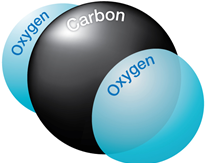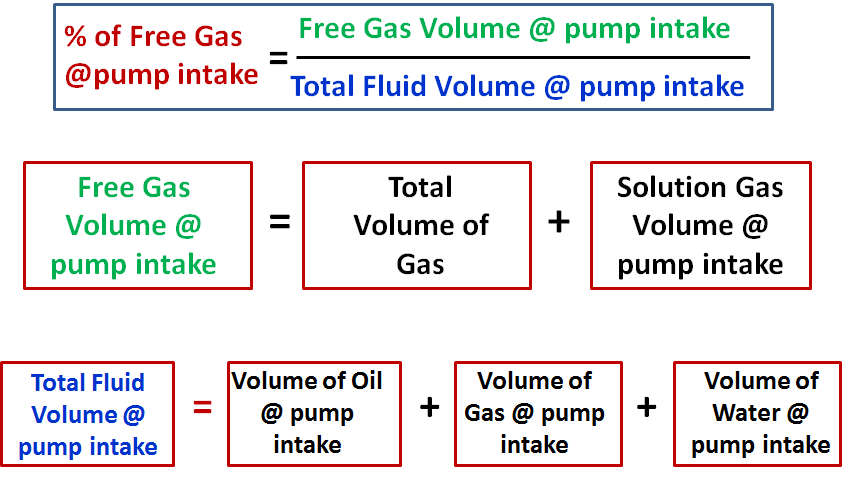## Effect of CO2 on downhole flowrate calculation

Downhole flow rate can be calculated from surface flow rate (stock tank barrels) using the following equation. It is assumed that no gas is dissolved in the water phase and the water formation volume factor is equal to one.

Downhole flow rate = [(Oil rate)sc × Bo] + [(Free GOR) × (Oil rate)sc × Gas FVF] + (Water rate)sc

Free GOR = Producing GOR – Solution GOR, therefore:

## q = ( Qo × Bo ) + [ ( R – Rs) × Qo × Bg × 1000] + Qw

Where:

• q = downhole flow rate (bbl/d or m3/d)
• Qo = Oil flow rate at standard conditions (stb/d or m3sc/d)
• Bo = Oil formation volume factor (bbl/stb or m3sc/m3sc)
• R = Producing gas-oil ratio (scf/stb or m3sc/m3sc)
• Rs = Solution gas-oil ration (scf/stb or m3sc/m3sc)
• Bg = Gas formation vol. factor (bbl/mscf or m3sc/m3sc)
• Qw = Water flow rate at standard conditions (stb/d or m3sc/d)

### Effect of CO2 on downhole flowrate calculation:If CO2 is present, the calculation of downhole flow rate becomes more complex for many reasons:

## ESP design – Step 3: Gas Calculations

The presence of free gas in the tubing above the pump changes the fluid density, consequently reduces the required discharge pressure. Also the performance of centrifugal pumps is considerably affected by the presence of free gas in the pumped fluid. The pump starts producing lower than normal head as the produced GLR (Gas to Liquid Ratio) at the pumping conditions increases beyond a critical value. The critical value of the ratio or percentage of free gas present at the pump intake to the total volume of fluid depends on the pump impeller design (typical critical values are shown in the article “ESP: Gas handling device “). Therefore, it is essential to determine the percentage of free gas by volume at the pumping conditions in order select the proper pump and gas handling device (if required).

### Percentage of free gas by volume:

Assuming that Solution GOR (Rs), Gas Volume Factor (Bg) and Oil Formation Volume Factor (Bo) are known, the total volume of fluids and the percentage of free gas released at the pump intake should be calculated.